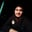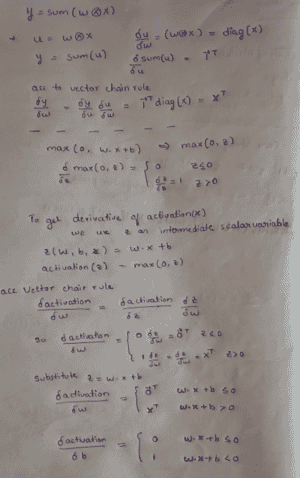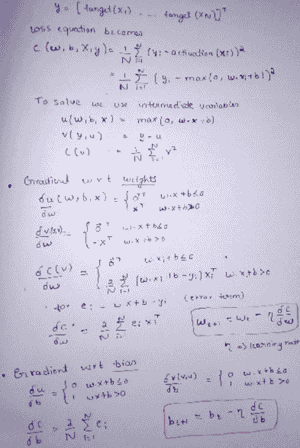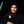Trusted answers to developer questions
Trusted Answers to Developer Questions

Related Tags

community creator
deep learning
calculus

# Gradient Calculation in Matrix Calculus for Deep LearningKiran U Kamath

Grokking Modern System Design Interview for Engineers & Managers

Ace your System Design Interview and take your career to the next level. Learn to handle the design of applications like Netflix, Quora, Facebook, Uber, and many more in a 45-min interview. Learn the RESHADED framework for architecting web-scale applications by determining requirements, constraints, and assumptions before diving into a step-by-step design process.In the two previous shots we have looked into the Jacobian matrix, element wise operations, derivatives involving single expressions and vector sum reduction, and the chain rule. You can look through that in parts 1 and 2.

## The gradient of neuron activation

Let us compute the derivative of a typical neuron activation for a single neural network computation unit with respect to the model parameters, w and b.

activation(x) = max(0, w . x + b)

This represents a neuron with fully connected weights and relu. Let us compute the derivative of ( w . x + b ) wrtwith respect to w and b\ dot product w. x is the summation of the element-wise multiplication of elements. The partial derivative of sum(w ⊗ x) can be calculated using the chain rule with the intermediate vector variable.The above image uses the max(0, z) function call on scalar z, which just says to treat all negative z values as 0. The derivative of the max function is a piecewise function. When z ≤ 0, the derivative is 0 because z is a constant. When z > 0, the derivative of the max function is just the derivative of z, which is 1.

When the activation function clips affine function output z to 0, the derivative is 0 with respect to any weight w i. When z > 0, it’s as if the max function disappears and we just get the derivative of z with respect to the weights.

## The gradient of the neural network loss function

Training a neuron requires that we take the derivative of our loss or “cost” function with respect to the parameters of our model, w and b.

We need to calculate gradient wrt weights and bias.

Let X = [x 1 , x 2 , … , xN ] T (T means transpose)If the error is 0, then the gradient is 0 and we have arrived at the minimum loss. If ei is some small positive difference, the gradient is a small step in the direction of x. If e 1 is large, the gradient is a large step in that direction. We want to reduce, not increase, the loss, which is why the gradient descent recurrence relation takes the negative of the gradient to update the current position.

Look at things like the shape of a vector (long or tall), if it’s a variable scalar or a vector, and the dimensions of the matrix. Vectors are represented by bold letters. After reading this shot, please read this paper to get a better understanding. The paaper has unique way of explaining concepts, that goes from simple to complex. When we reach the end of paper, we will solve it ourselves since we have a deep understanding of simple expressions.

First, we start with functions of simple parameters represented by f(x). Then, we move to functions of the form f(x,y,z). To calculate the derivatives of such functions, we use partial derivatives that are calculated with respect to specific parameters. Next, we move to the scalar function of a vector of input parameters as f(x), wherein the partial derivatives of f(x) are represented as vectors. Lastly, we see that f(x) represents a set of scalar functions of the form f(x).

You have reached the end of a 3 part series. If you missed parts 1 and 2, visit them now.

RELATED TAGS

community creator
deep learning
calculus

CONTRIBUTORKiran U Kamath